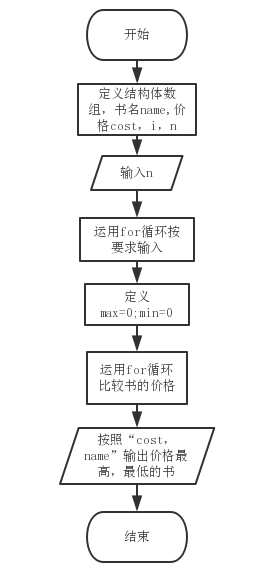## 第三次作业

2018-04-23 20:39  史晨宇  阅读(178)  评论(2编辑  收藏  举报

1 完成PTA结构部分编程1和2，采用函数编程3
2 课上疑点：讨论查阅分析  struct sk{int a; char *str)}*p;   p->str++ 中的++ 加向________?  答：p中字符指针str

1 设计思路（6分）
(1)主要描述题目算法（1分）。可以用文字描述（不允许使用伪代码），如：

(2)流程图（4分）2.实验代码（2分）

#include<stdio.h>
#include<string.h>
#include<stdlib.h>
struct book
{
char name;
double cost;
};
main()
{
struct book s;
int i,n;
scanf("%d",&n);
for(i=0;i<n;i++)
{
scanf("\n");
gets(s[i].name);
scanf("%lf",&s[i].cost);
}
int max=0,min=0;
for(i=1;i<n;i++)
{
if(s[i].cost>s[max].cost){max=i;}
if(s[i].cost<s[min].cost){min=i;}
}
printf("%.2f, %s\n",s[max].cost,s[max].name);
printf("%.2f, %s",s[min].cost,s[min].name);

}

3.本题调试过程碰到问题及解决办法（12分）

错误原因：未调用数据库这个玩意  #include<string.h>

改正方法：照着同学加上的

1 设计思路（6分）
(1)主要描述题目算法（1分）。、

2.实验代码（2分）

#include<stdio.h> #include<stdlib.h> #include<string.h>
struct score{
char num;
char name;
double s;
};
int main(){
struct score s;
int i,n;
double sum=0,average;
scanf("%d",&n);
for(i=0;i<n;i++){
scanf("%s%s%lf",&s[i].num,&s[i].name,&s[i].s);
}
for(i=0;i<n;i++){
sum=sum+s[i].s;
}
average=sum/n;
printf("%.2f\n",average);
for(i=0;i<n;i++){
if(s[i].s<average){
printf("%s %s\n",s[i].name,s[i].num);
}
}
return 0;
}

3.本题调试过程碰到问题及解决办法（12分）

错误原因：未调用数据库这个玩意  #include<string.h>

改正方法：照着同学加上的